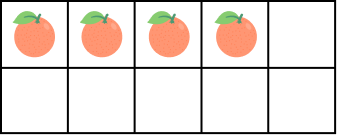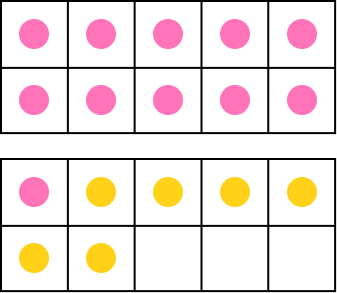# Make a Ten Strategy for Addition – Chart, Ten Frames, Examples

Home » Math-Vocabluary » Make a Ten Strategy for Addition – Chart, Ten Frames, Examples

## What Is the “Make a Ten Strategy” in Math?

The “make a ten Strategy” or the “making ten strategy” is a mathematical strategy where you add numbers to make a sum of ten.

The “making 10 strategy” involves changing one of the numbers in an Addition problem into the number 10 in order to make the calculation easier.

Example: Let’s find 7 + 6.

Adding 3 to 7 will make it 10.

7 + 3 = 10.

This 3 comes from the second number, 6.

So, 6 – 3 = 3.

Now, we can represent this as

7 + 6 = (7 + 3) + 3 = 10 + 3 = 13

Thus, 7 + 6 = 13.

It is a very useful strategy to help kids understand basic addition, compensation strategy, and number sense. It is also called friends of 10, making ten, making 10, make-10, different ways to make 10, or combinations to ten.

Dealing with the number 10 is always easy. We always rely on our 10 fingers when counting or when taking away one number from another. As we use the decimal number system (number system with base 10), the number 10 has a very significant role in all the arithmetic operations that we carry out on numbers.

## Making Ten Chart

Here’s a chart that displays number pairs adding to ten. This will help in solving problems using the making ten strategy.

Adding numbers by making 10 involves identifying pairs of numbers that add up to 10 and using them to simplify the addition problem.

## What Is a Ten Frame?

A ten frame is a 2 × 5 rectangular frame with ten equal squares. It is a math tool that consists of a grid with two rows, each containing five squares. It is particularly useful for representing numbers up to ten.

• Why Do We Use Ten Frames?

Ten frames are two-by-five rectangular grids, where objects like counters can be placed to represent numbers ranging from 0 to 10 or less.

Ten frames make it easier to understand the “making ten” strategy. Ten frames are often used in conjunction with the “Making 10” strategy to help students visualize and understand how to make ten.

In the context of the “making 10 strategy,” ten frames can be used to represent the numbers being added and to visualize how to combine them to make ten.

In essence, ten frames serve as a visual aid to support the “making 10 strategy,” making it a valuable teaching tool.

• How to Draw a Ten Frame

Let’s discuss two examples to understand how to draw a ten frame to represent and understand the given addition fact.

Example 1: 3 + 7 = 10

Draw a 2 × 5 rectangular grid as shown below.

Draw 3 circles or symbols in three squares of the ten frame.

How many dots are needed to make 10?

Count the number of empty squares. There are 7 empty positions.

7 more circles are needed to fill 10 positions.

Thus, 3  +  7 = 10

Example 2: Suppose we have 4 marbles. How many more marbles are needed to make 10 marbles?

Draw 4 circles representing 4 marbles.

Count the number of spaces that are remaining. In this case, the number of spaces with no symbols is 6.

So, the number needed to make a ten is 6

4 + 6 = 10.

• Applications of Ten Frames

Ten frames make it easy to add numbers. It is also used in counting. By using ten frames, we are able to develop the knowledge of tens and ones as one ten frame means tens.

Examples:

i) Four ten frames = 4 × 10 = 40

ii) 3 ten frames + 7 squares = 3 tens + 7 ones = 30 + 7 = 37

Suppose we have to add the numbers 7 and 6.

In simple words, we have to evaluate 7 + 6.

Draw two ten frames.

Place 7 chips in the first ten-frame and 6 chips in the second ten-frame. We get:

In the top ten-frame, 3 boxes are empty. So, we will take 3 chips from the bottom ten frame and will put them in the top ten frame. This will fill the first frame completely. In the second frame, there are 3 chips.

We write this as

7 + 6 = (7 + 3) + 3 = 10 + 3 = 13

• Using ten frames for subtraction

Let’s understand this with the help of an example.

Example 1: Sophie has 12 caps. She gave 5 caps to her sister. How many caps are left?

Number of caps left with Sophie = 12 – 5

Draw two ten frames.

Draw 12 Pink circles using two ten frames. Since we wish to subtract 5, replace the first 5 circles by Blue colored circles, or you can cross off the first 5 circles.

Now, count the number of Pink circles left.

There are 7 Pink circles left.

Thus, 12 – 5 = 7

Therefore, 7 caps are left with Sophie.

## Making Ten Strategy for Addition

The make ten strategy makes dealing with addition of large numbers easier. Here, we try to manipulate and change one of the addends into the number 10 (or a multiple of 10).

• Adding one-digit numbers using ten strategy

One digit numbers are 0, 1, 2, 3, 4, 5, 6, 7, 8, and 9.

Applying the make-10 strategy on single-digit numbers is simple.

Example 1: 9 + 4

We know that 9 + 1 = 10.

Take 1 away from 4 and add it to 9.

9 + 4 = 9 + (1 + 3) = (9 + 1) + 3 = 10 + 3 = 13

Example 2: 8 + 7

We know that 8 + 2 = 10.

Take 2 away from 7 and add it to 8.

8 + 7 = 8 + (2 + 5) = (8 + 2) + 5 = 10 + 5 = 15

• Adding two-digit numbers using the make ten strategy

Two digit numbers are the numbers in the range 10 to 99 (both 10 and 99 included). The making ten strategy is not limited to the number 10. We can also extend it to multiples of ten such as 20, 30, 40, and so on.

Example: Mike bought a book for $\$47$and a pen for$\$15$. How much money did he spend in all?

Money spent by Mike $= \$47 + \$15$

Let us find the relation between 7 and 5.

7 + 3 = 10.

Take 3 away from 15 and add it to 47.

47 + 15 = 47 + 3 + 12 = 50 + 12 = 62

Mike spent $62. Example 2: 54 + 27 4 + 6 = 10. Take 6 away from 27 and add it to 54. 54 + 27 = 54 + 6 + 21 = 60 + 21 = 81 ## Making Ten Strategy for Subtraction We can also use the make ten strategy to subtract two numbers. Example 1: 14 – 8 We know that 14 – 4 = 10 We will write 8 as 8 = 4 + 4 14 – 8 = 14 – (4 + 4) = 14 – 4 – 4 = 10 – 4 = 6 ## Facts about the Making Ten Strategy • The making ten strategy is a mental math approach used to simplify addition problems. • Ten frames are often used as visual aids to help students grasp the concept of Making Ten. ## Conclusion In this article, we learned about the Making Ten strategy, a helpful technique in basic arithmetic. By breaking down numbers to create sums of ten, we can simplify calculations and enhance mental math skills. Let’s solidify our understanding by working through examples and practicing MCQs for better mastery. ## Solved Examples on Making Ten Strategy 1. How many more apples are needed to make ten apples? Solution: There are 3 apples. Number of empty squares = 7 7 more apples are needed to make ten apples. 2. Write the addition sentence represented by the given ten frames. Solution: There are 8 red chips and 9 yellow chips. Total number of chips = 17 So, the addition sentence represented by the given ten frames is 8 + 9 = 17 3. Shyna has 5 pencils. Her father gave her 9 more pencils. Find the total number of pencils using the ten strategy. Solution: Number of pencils with Shyna = 5 Number of pencils her father gave to her = 9 Number of pencils with Shyna now = 5 + 9 = 4 + 1 + 9 = 4 + 10 = 14 4. Sam had$15. He gave $8 to his sister. How much money is left with him? Solution: Amount of money with Sam$= \$15$

Amount of money he gave to her sister $= \$8$Amount of money left with him$= \$15 – \$8$The related fact is$\$8 +$ ? $= \$15$The number of yellow chips to make 15 is 7. Amount left with Sam$= \$7$.

5. Sofia has 34 oranges. She wants to take 55 oranges to her grandmother’s house. How many more oranges will she buy using the ten strategy?

Solution:

Number of oranges Sofia has = 34

Number of oranges she wants = 55

Number of oranges she will buy = 55 – 34

55 – 34 = 55 – 30 – 29 = 50 – 29 = 21

## Practice Problems on Making Ten Strategy

1

### How many tomatoes are needed to make a ten in the below ten frame?4
5
6
7
CorrectIncorrect
Number of empty squares = 6
So, 4 + 6 = 10
2

### Which addition sentence is represented in the following ten frame?3 + 7 = 10
3 + 6 = 10
4 + 6 = 10
4 + 5 = 10
CorrectIncorrect
Correct answer is: 3 + 7 = 10
There are 3 chips. There are 7 empty squares.
So, 3 + 7 = 10.
3

### How do you find 15 – 6 using the making ten strategy?

15 – 2 – 4
15 – 5 – 1
15 – 4 – 2
12 + 3 – 6
CorrectIncorrect
Correct answer is: 15 – 5 – 1
6 = 5 + 1
15 – 6 = 15 – (5 + 1) = 15 – 5 – 1
4

### Which subtraction sentence is represented in the given ten frame?17 – 11 = 6
17 – 12 = 5
18 – 11 = 7
18 – 12 = 6
CorrectIncorrect
Correct answer is: 17 – 11 = 6
There are 17 chips in total.
There are 11 red chips and 6 yellow chips.
So, 17 – 11 = 6.
5

### How many chips are needed to make 10 in the given ten frame?2
3
4
5
CorrectIncorrect